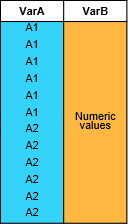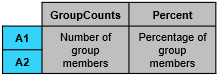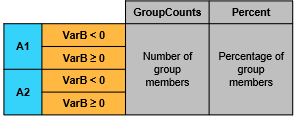# groupcounts

Number of group elements

Since R2019a

## Syntax

``G = groupcounts(T,groupvars)``
``G = groupcounts(T,groupvars,groupbins)``
``G = groupcounts(___,Name,Value)``
``B = groupcounts(A)``
``B = groupcounts(A,groupbins)``
``B = groupcounts(___,Name,Value)``
``[B,BG,BP] = groupcounts(A,___)``

## Description

### Table Data

example

````G = groupcounts(T,groupvars)` returns the unique grouping variable combinations for table or timetable `T`, the number of members in each group, and the percentage of the data each group represents in the range [0, 100]. Groups are defined by rows in the variables in `groupvars` that have the same unique combination of values. Each row of the output table corresponds to one group. For example, ```G = groupcounts(T,"HealthStatus")``` returns a table with the count and percentage of each group in the variable `HealthStatus`.For more information, see Group Counts Computation.```

example

````G = groupcounts(T,groupvars,groupbins)` specifies to bin rows in `groupvars` according to binning scheme `groupbins` prior to grouping. For example, ```G = groupcounts(T,"SaleDate","year")``` returns the group counts and group percentages for all sales in `T` within each year according to the grouping variable `SaleDate`.```

example

````G = groupcounts(___,Name,Value)` specifies additional grouping properties using one or more name-value arguments for any of the previous syntaxes. For example, ```G = groupcounts(T,"Category1","IncludeMissingGroups",false)``` excludes the group made from missing data of type `categorical` indicated by `<undefined>` in `Category1`.```

### Array Data

````B = groupcounts(A)` returns the number of members in each group in vector, matrix, or cell array `A`. Groups are defined by rows in the column vectors in `A` that have the same unique combination of values. Each row of `B` contains the count for one group.```
````B = groupcounts(A,groupbins)` specifies to bin the data according to binning scheme `groupbins` prior to grouping.```
````B = groupcounts(___,Name,Value)` specifies additional grouping properties using one or more name-value arguments for either of the previous syntaxes for an input array.```

example

````[B,BG,BP] = groupcounts(A,___)` returns additional group information. `BG` is the unique grouping vector combinations corresponding to the rows in `B`. `BP` is the percentage of the data each group count in `B` represents. The percentages are in the range [0, 100].```

## Examples

collapse all

Compute the number of elements in each group based on table data.

Create a table `T` that contains information about eight individuals.

```HealthStatus = categorical(["Poor"; "Good"; "Fair"; "Fair"; "Poor"; "Excellent"; "Good"; "Excellent"]); Smoker = logical([1; 0; 0; 1; 1; 0; 0; 1]); Weight = [176; 153; 131; 133; 119; 120; 140; 129]; T = table(HealthStatus,Smoker,Weight)```
```T=8×3 table HealthStatus Smoker Weight ____________ ______ ______ Poor true 176 Good false 153 Fair false 131 Fair true 133 Poor true 119 Excellent false 120 Good false 140 Excellent true 129 ```

Group the individuals by health status, and return the number of and percentage of individuals in each group.

`G1 = groupcounts(T,"HealthStatus")`
```G1=4×3 table HealthStatus GroupCount Percent ____________ __________ _______ Excellent 2 25 Fair 2 25 Good 2 25 Poor 2 25 ```

Group the individuals by health status and smoker status, and return the number of and percentage of individuals in each group. By default, `groupcounts` suppresses groups with zero elements, so some unique combinations of the grouping variable values are not returned.

`G2 = groupcounts(T,["HealthStatus","Smoker"])`
```G2=6×4 table HealthStatus Smoker GroupCount Percent ____________ ______ __________ _______ Excellent false 1 12.5 Excellent true 1 12.5 Fair false 1 12.5 Fair true 1 12.5 Good false 2 25 Poor true 2 25 ```

To return a row for each group, including those with zero elements, specify `IncludeEmptyGroups` as `true`.

`G3 = groupcounts(T,["HealthStatus","Smoker"],"IncludeEmptyGroups",true)`
```G3=8×4 table HealthStatus Smoker GroupCount Percent ____________ ______ __________ _______ Excellent false 1 12.5 Excellent true 1 12.5 Fair false 1 12.5 Fair true 1 12.5 Good false 2 25 Good true 0 0 Poor false 0 0 Poor true 2 25 ```

Group data according to specified bins.

Create a timetable containing sales information for days within a single month.

```TimeStamps = datetime([2017 3 4; 2017 3 2; 2017 3 15; 2017 3 10; ... 2017 3 14; 2017 3 31; 2017 3 25; ... 2017 3 29; 2017 3 21; 2017 3 18]); Profit = [2032 3071 1185 2587 1998 2899 3112 909 2619 3085]'; ItemsSold = [14 13 8 5 10 16 8 6 7 11]'; TT = timetable(TimeStamps,Profit,ItemsSold)```
```TT=10×2 timetable TimeStamps Profit ItemsSold ___________ ______ _________ 04-Mar-2017 2032 14 02-Mar-2017 3071 13 15-Mar-2017 1185 8 10-Mar-2017 2587 5 14-Mar-2017 1998 10 31-Mar-2017 2899 16 25-Mar-2017 3112 8 29-Mar-2017 909 6 21-Mar-2017 2619 7 18-Mar-2017 3085 11 ```

Compute the group counts by the total items sold, binning the groups into intervals of item numbers.

`G = groupcounts(TT,"ItemsSold",[0 4 8 12 16])`
```G=3×3 table disc_ItemsSold GroupCount Percent ______________ __________ _______ [4, 8) 3 30 [8, 12) 4 40 [12, 16] 3 30 ```

Compute the group counts binned by day of the week.

`G = groupcounts(TT,"TimeStamps","dayname")`
```G=5×3 table dayname_TimeStamps GroupCount Percent __________________ __________ _______ Tuesday 2 20 Wednesday 2 20 Thursday 1 10 Friday 2 20 Saturday 3 30 ```

Determine which elements in a vector appear more than once.

Create a column vector with values between 1 and 5.

`A = [1 1 2 2 3 5 3 3 1 4]';`

Determine the unique groups in the vector and count the group members.

`[B,BG] = groupcounts(A)`
```B = 5×1 3 2 3 1 1 ```
```BG = 5×1 1 2 3 4 5 ```

Determine which elements in the vector appear more than once by creating a logical index for the groups with a count larger than 1. Index into the groups to return the vector elements that are duplicated.

`duplicates = BG(B > 1)`
```duplicates = 3×1 1 2 3 ```

Compute the group counts for a set of people grouped by their health status and smoker status.

Store information about eight individuals as three vectors of different types.

```HealthStatus = categorical(["Poor"; "Good"; "Fair"; "Fair"; "Poor"; "Excellent"; "Good"; "Excellent"]); Smoker = logical([1; 0; 0; 1; 1; 0; 0; 1]); Weight = [176; 153; 131; 133; 119; 120; 140; 129];```

Grouping by health status and smoker status, compute the group counts. Specify three outputs to also return the groups `BG` and group count percentages `BP`.

`BG` is a cell array containing two vectors that describe the groups as you look at their elements row-wise. For instance, the first row of `BG{1}` indicates that the individuals in the first group have a health status `Excellent`, and the first row of `BG{2}` indicates that they are nonsmokers. Finally, `BP` contains the percentage of members in each group for the corresponding groups in `BG`.

```[B,BG,BP] = groupcounts({HealthStatus,Smoker},"IncludeEmptyGroups",true); B```
```B = 8×1 1 1 1 1 2 0 0 2 ```
`BG{1}`
```ans = 8x1 categorical Excellent Excellent Fair Fair Good Good Poor Poor ```
`BG{2}`
```ans = 8x1 logical array 0 1 0 1 0 1 0 1 ```
`BP`
```BP = 8×1 12.5000 12.5000 12.5000 12.5000 25.0000 0 0 25.0000 ```

## Input Arguments

collapse all

Input table, specified as a table or timetable.

Input array, specified as a column vector, group of column vectors stored as a matrix, or cell array of column vectors, character row vectors, or matrices.

Grouping variables or vectors, specified as one of the options in this table. For table or timetable input data, `groupvars` indicates which variables to use to compute groups in the data. Other variables not specified by `groupvars` are not operated on and do not pass through to the output.

Indexing SchemeExamples

Variable names:

• A string, character vector, or cell array

• A `pattern` object

• `"A"` or `'A'` — A variable named `A`

• `["A","B"]` or `{'A','B'}` — Two variables named `A` and `B`

• `"Var"+digitsPattern(1)` — Variables named `"Var"` followed by a single digit

Variable index:

• An index number that refers to the location of a variable in the table

• A vector of numbers

• A logical vector. Typically, this vector is the same length as the number of variables, but you can omit trailing `0` or `false` values

• `3` — The third variable from the table

• `[2 3]` — The second and third variables from the table

• `[false false true]` — The third variable

Function handle:

• A function handle that takes a table variable as input and returns a logical scalar

• `@isnumeric` — All the variables containing numeric values

Variable type:

• `vartype("numeric")` — All the variables containing numeric values

Example: `groupcounts(T,"Var3")`

Binning scheme for grouping variables or vectors, specified as one or more of the following binning methods. To apply the same binning method to all grouping variables or vectors, specify one binning method. To apply a different binning method to each grouping variable or vector, specify a cell array of binning methods, where each cell contains the binning method for the corresponding grouping variable or vector.

• `"none"` — No binning.

• Vector of bin edges — The bin edges define the bins. You can specify the edges as numeric values or as `datetime` values for `datetime` grouping variables or vectors.

• Number of bins — The number determines how many equally spaced bins to create. You can specify the number of bins as a positive integer scalar.

• Length of time (bin width) — The length of time determines the width of each bin. You can specify the bin width as a `duration` or `calendarDuration` scalar for `datetime` or `duration` grouping variables or vectors.

• Name of time unit (bin width) — The name of the time unit determines the width of each bin. You can specify the bin width as one of the options in this table for `datetime` or `duration` grouping variables or vectors.

ValueDescriptionData Type
`"second"`

Each bin is 1 second.

`datetime` and `duration`
`"minute"`

Each bin is 1 minute.

`datetime` and `duration`
`"hour"`

Each bin is 1 hour.

`datetime` and `duration`
`"day"`

Each bin is 1 calendar day. This value accounts for daylight saving time shifts.

`datetime` and `duration`
`"week"`Each bin is 1 calendar week.`datetime` only
`"month"`Each bin is 1 calendar month.`datetime` only
`"quarter"`Each bin is 1 calendar quarter.`datetime` only
`"year"`

Each bin is 1 calendar year. This value accounts for leap days.

`datetime` and `duration`
`"decade"`Each bin is 1 decade (10 calendar years).`datetime` only
`"century"`Each bin is 1 century (100 calendar years).`datetime` only
`"secondofminute"`

Bins are seconds from 0 to 59.

`datetime` only
`"minuteofhour"`

Bins are minutes from 0 to 59.

`datetime` only
`"hourofday"`

Bins are hours from 0 to 23.

`datetime` only
`"dayofweek"`

Bins are days from 1 to 7. The first day of the week is Sunday.

`datetime` only
`"dayname"`Bins are full day names, such as `"Sunday"`.`datetime` only
`"dayofmonth"`Bins are days from 1 to 31.`datetime` only
`"dayofyear"`Bins are days from 1 to 366.`datetime` only
`"weekofmonth"`Bins are weeks from 1 to 6.`datetime` only
`"weekofyear"`Bins are weeks from 1 to 54.`datetime` only
`"monthname"`Bins are full month names, such as `"January"`.`datetime` only
`"monthofyear"`

Bins are months from 1 to 12.

`datetime` only
`"quarterofyear"`Bins are quarters from 1 to 4.`datetime` only

Example: `G = groupcounts(T,"Var1",[-Inf 0 Inf])`

Example: ```G = groupcounts(T,["Var1" "Var2"],{"none" "year"})```

### Name-Value Arguments

Specify optional pairs of arguments as `Name1=Value1,...,NameN=ValueN`, where `Name` is the argument name and `Value` is the corresponding value. Name-value arguments must appear after other arguments, but the order of the pairs does not matter.

Example: ```G = groupcounts(T,groupvars,groupbins,IncludedEdge="right")```

Before R2021a, use commas to separate each name and value, and enclose `Name` in quotes.

Example: ```G = groupcounts(T,groupvars,groupbins,"IncludedEdge","right")```

Included bin edge for binning scheme, specified as either `"left"` or `"right"`, indicating which end of the bin interval is inclusive.

You can specify `IncludedEdge` only if you also specify `groupbins`, and the value applies to all binning methods for all grouping variables or vectors.

Option to treat missing values as a group, specified as a numeric or logical `1` (`true`) or `0` (`false`). If `IncludeMissingGroups` is `true`, then `groupcounts` treats missing values, such as `NaN`, in a grouping variable or vector as a group. If a grouping variable or vector has no missing values, or if `IncludeMissingGroups` is `false`, then `groupcounts` does not treat missing values as a group.

Option to include empty groups in the group counts operation, specified as a numeric or logical `0` (`false`) or `1` (`true`). If `IncludeEmptyGroups` is `false`, then `groupcounts` omits empty groups. If `IncludeEmptyGroups` is `true`, then `groupcounts` includes empty groups.

An empty group occurs in these cases:

• A possible value of a grouping variable or vector is not represented in the input data, such as in a categorical, logical, or binned numeric variable or vector. For example, if no row in the input table has a value of `true` for a logical grouping variable, then `true` defines an empty group.

• A unique combination of grouping variables or vectors is not represented in the input data. For example, if there is no row in the input table where the value of grouping variable `A` is `A1` and the value of grouping variable `B` is `B1`, then `A1_B1` defines an empty group.

## Output Arguments

collapse all

Output table for table or timetable input data, returned as a table. `G` contains the computed groups, number of elements in each group, and percentages represented by each group count. For a single grouping variable, the output groups are sorted according to the order returned by the `unique` function with the `"sorted"` option.

Group counts for array input data, returned as a column vector. `B` contains the number of elements in each group.

Groups for array input data, returned as a column vector or cell array of column vectors. For a single grouping vector, the output groups are sorted according to the order returned by the `unique` function with the `"sorted"` option.

For more than one input vector, `BG` is a cell array containing column vectors of equal length. Information for each group is contained in the elements of a row across all vectors in `BG`. Each group maps to the corresponding row of the output array `B`.

Group count percentages for array input data, returned as a column vector. `BP` contains a percentage in the range `[0, 100]` for each group in `B`.

collapse all

### Group Counts Computation

This table illustrates group counts computations.

Sample Table `T`Syntax ExampleResulting Table`groupcounts(T,"VarA")``groupcounts(T,["VarA" "VarB"],{"none",[-Inf 0 Inf]})`## Tips

• When making many calls to `groupcounts`, consider converting grouping variables to type `categorical` or `logical` when possible for improved performance. For example, if you have a string array grouping variable (such as `HealthStatus` with elements `"Poor"`, `"Fair"`, `"Good"`, and `"Excellent"`), you can convert it to a categorical variable using the command `categorical(HealthStatus)`.

## Version History

Introduced in R2019a

expand all